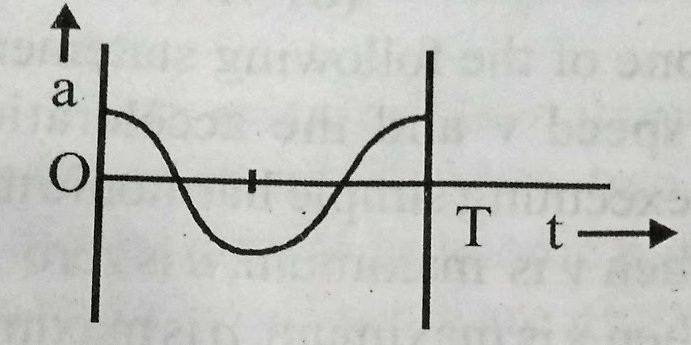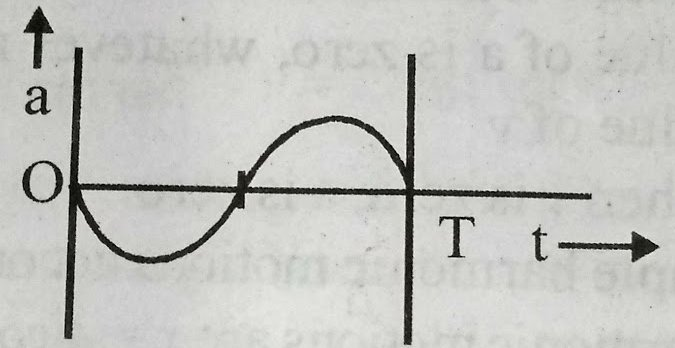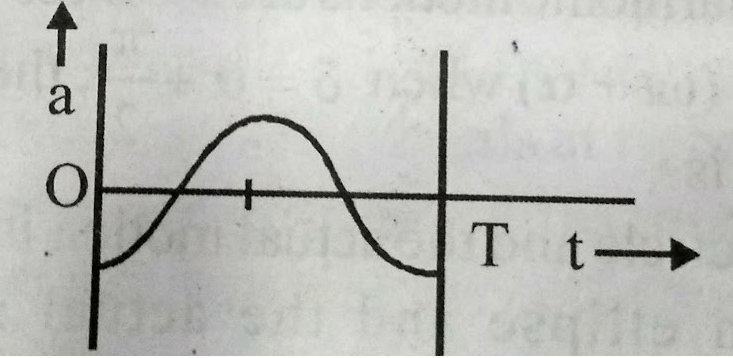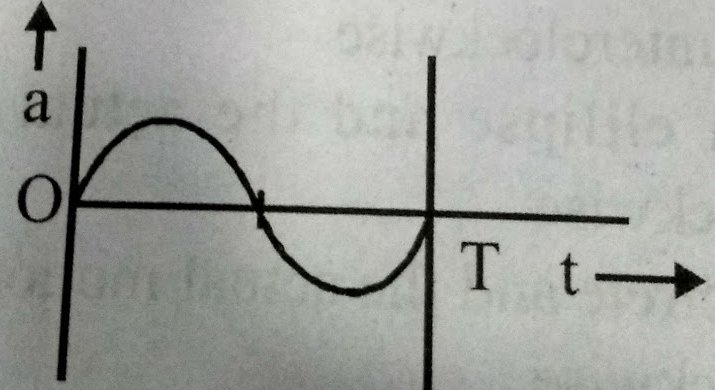## Filters

Sort by :
Clear All
Q
Medical
113 Views   |

The oscillation of a body on a smooth horizontal surface is represented by the equation,

X = A cos ( t)

where, X = displacement at time t

= frequency of oscillation

Which one of the following graphs shows correctly the variation of 'a' with 't'?

• Option 1)• Option 2)• Option 3)• Option 4)As discussed Composition of two SHM in perpendicular direction -     - wherein Resultant equation    a=A coswt v=-AwSinwt a= i.e the variation of a with and correctly shown by graph (3) Option 1) This option is incorrect  Option 2) This option is incorrect  Option 3) This option is correct  Option 4) This option is incorrect
Medical
88 Views   |

The number of possible natural oscillation of air column in a pipe closed at one end of length 85 cm whose frequencies lie below 1250 Hz are:

( velocity of sound = 340 ms-1)

• Option 1)

4

• Option 2)

5

• Option 3)

7

• Option 4)

6

As learnt  Closed organ pipe - A closed organ pipe is a cylindrical tube having an air colume with one end closed. - wherein Condition of constructive interference         f =  if    n = 0     f0 = 100 Hz      n = 1      f1 = 300 Hz      n = 2      f2 = 500 Hz      n = 3      f3 = 700 Hz      n = 4      f4 = 900 Hz      n = 5      f5 = 1100 Hz      n = 6      f6 = 1300 Hz Possible natural...
Medical
112 Views   |

A speeding motorcyclist sees traffic jam ahead of him. He slows down to 36 km/hour. He finds that traffic has eased and a car moving a head of him at 18 km/hour is honking at a frequency of 1392 Hz. If the speed of sound is 343 m/s, the frequency of the honk as heard by him will be:

• Option 1)

1332 Hz

• Option 2)

1372 Hz

• Option 3)

1412 Hz

• Option 4)

1464 Hz

As we discussed frequency of sound when source is moving away and observer is moving towards the source - - wherein Speed of sound Speed of observer speed of source Original frequency apparent frequency   According to doppler's effect   Option 1) 1332 Hz Incorrect Option 2) 1372 Hz Incorrect Option 3) 1412 Hz Correct Option 4) 1464 Hz Incorrect
Exams
Articles
Questions